# Nature of Physical Laws

#### notes

NATURE OF PHYSICAL LAWS

How Do Physical Laws Come into Existence?

Our universe is ordered in an intelligible way. Physicists explore the universe. The range of their exploration is extremely wide: particles that are smaller than the atoms in size to the stars that are very far away. In addition to finding facts by observations and experimentation, physicists attempt to discover the laws that summarize (often as mathematical equations) these facts. When a physical phenomenon governed by the different types of forces takes place several quantities change with time but some special quantities remain constant and such observation leads to the origin of conservative principles in Physics.

Example:- Suppose a ball is freely falling to the ground. Some quantities such as velocity, displacement etc. change with time but mechanical energy (the sum of kinetic and potential energy) remains constant. And that's when we say mechanical energy is conserved under free fall.
Free fall means the force acting on the ball is the only gravitational force, however in real conditions, air resistance also play a big role that is a type of friction force.

The example we studied follows the following general statement:
“For motion under an external conservative force, the total mechanical energy of a body is a constant.”
This law restricted for a conservative force should not be confused with the general law of conservation of energy of an isolated system (which is the First Law of Thermodynamics).

Conservation Laws in Physics :-

The physical quantities that remain unchanged in a process are called conserved quantities. Some of the general conservation laws in nature include the laws of conservation of energy, mass, linear momentum, angular momentum, charge, parity, etc.

Conservation of energy implies that energy can be neither created nor destroyed, although it can be changed from one form (mechanical, kinetic, chemical, etc.) into another. In an isolated system the sum of all forms of energy therefore remains constant. For example, a falling body has a constant amount of energy, but the form of the energy changes from potential to kinetic. According to the theory of relativity, energy and mass are equivalent. Thus, the rest mass of a body may be considered a form of potential energy, part of which can be converted into other forms of energy.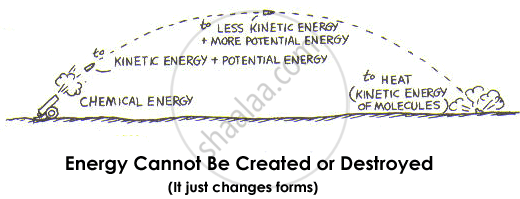Conservation of linear momentum expresses the fact that a body or system of bodies in motion retains its total momentum, the product of mass and velocity, unless an external force is applied to it. In an isolated system (such as the universe), there are no external forces, so momentum is always conserved. Because momentum is conserved, its components in any direction will also be conserved. Application of the law of conversation of momentum is important in the solution of collision problems. The operation of rockets exemplifies the conservation of momentum: the increased forward momentum of the rocket is equal but opposite in sign to the momentum of the ejected exhaust gases.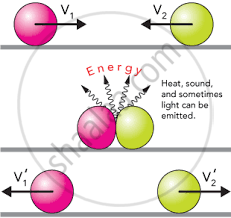Conservation of angular momentum of rotating bodies is analogous to the conservation of linear momentum. Angular momentum is a vector quantity whose conservation expresses the law that a body or system that is rotating continues to rotate at the same rate unless a twisting force, called a torque, is applied to it. The angular momentum of each bit of matter consists of the product of its mass, its distance from the axis of rotation, and the component of its velocity perpendicular to the line from the axis.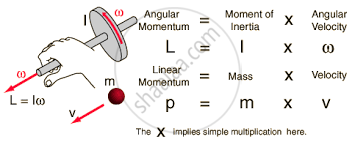Conservation of mass implies that matter can be neither created nor destroyed i.e., processes that change the physical or chemical properties of substances within an isolated system (such as conversion of a liquid to a gas) leave the total mass unchanged. Strictly speaking, mass is not a conserved quantity. However, except in nuclear reactions, the conversion of rest mass into other forms of mass-energy is so small that, to a high degree of precision, rest mass may be thought of as conserved.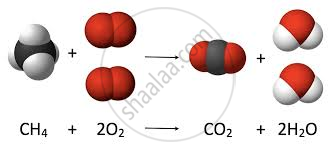Conversation of charge states that the total amount of electric charge in a system does not change with time. At a subatomic level, charged particles can be created, but always in pairs with equal positive and negative charge so that the total amount of charge always remains constant.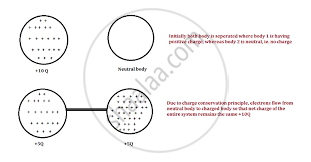If you would like to contribute notes or other learning material, please submit them using the button below.